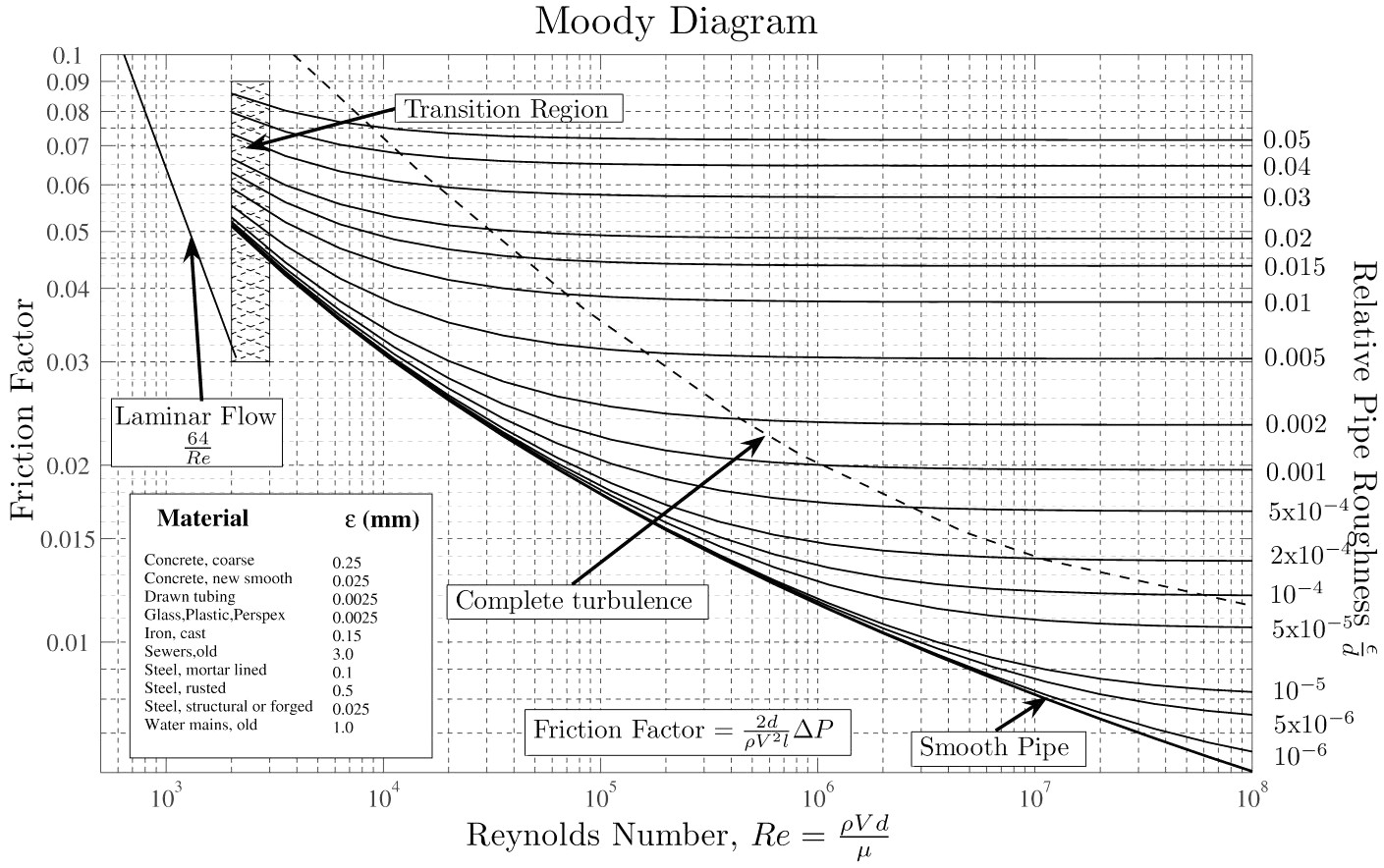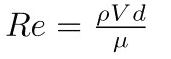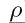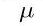# Moody DiagramUseful diagram for estimating the relative duct roughness factor in calculating pressure losses in ducts.

It uses the Reynolds Number (Re) a dimensionless number which is obtained fromwhereis the density of the air at temp and pressures prevailing andis the Viscosity of the air.  V is the velocity in the duct, d is the diameter of the duct.

Almost all LEV systems run with the Re above 2000 ie in the turbulent zone.